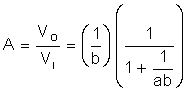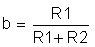SLOA011B January   2018  – July 2021

1. 1Introduction
2. 2Non-Inverting Amplifier
3. 3Inverting Amplifier
4. 4Simplified Op Amp Circuit Diagram
5. 5Op Amp Specifications
6. 6References
7. 7Glossary
8. 8Revision History

## 5.7 Large Signal Differential Voltage Amplification

Large signal differential voltage amplification, AVD, is the ratio of the output voltage change to the input differential voltage change, while holding VCM constant. This parameter is closely related to the open loop gain. The difference is that it is measured with an output load and therefore takes into account loading effects.

The DC value of AVD is published in the data sheet, but AVD is frequency dependent. Figure 5-12 shows a typical graph of AVD vs. frequency.

AVD is a design issue when precise gain is required. Consider equation Equation 16, where the loop gain of the non-inverting amplifier is given by:

Equation 33.where,

Equation 34.It is desired to control the gain of the circuit by selecting the appropriate resistors. The term 1/ab in the equation is seen as an error term. Unless a, or AVD, is large in comparison with 1/b, it will have an undesired effect on the gain of the circuit.